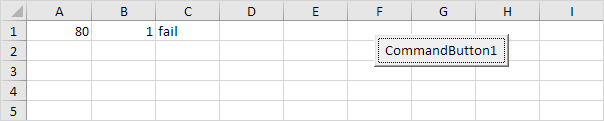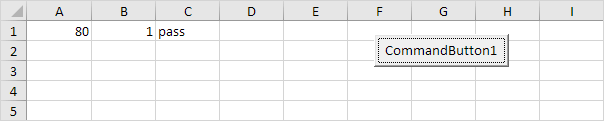# Logical Operators

The three most used logical operators in Excel VBA are: And, Or and Not. As always, we will use easy examples to make things more clear.

### Logical Operator And

Place a command button on your worksheet and add the following code lines:

Dim score1 As Integer, score2 As Integer, result As String

score1 = Range("A1").Value
score2 = Range("B1").Value

If score1 >= 60 And score2 > 1 Then
result = "pass"
Else
result = "fail"
End If

Range("C1").Value = result

Explanation: if score1 is greater than or equal to 60 and score2 is greater than 1, Excel VBA returns pass, else Excel VBA returns fail.

Result when you click the command button on the sheet:Conclusion: Excel VBA returns fail because score2 is not greater than 1.

### Logical Operator Or

Place a command button on your worksheet and add the following code lines:

Dim score1 As Integer, score2 As Integer, result As String

score1 = Range("A1").Value
score2 = Range("B1").Value

If score1 >= 60 Or score2 > 1 Then
result = "pass"
Else
result = "fail"
End If

Range("C1").Value = result

Explanation: if score1 is greater than or equal to 60 or score2 is greater than 1, Excel VBA returns pass, else Excel VBA returns fail.

Result when you click the command button on the sheet:Conclusion: Excel VBA returns pass because score1 is greater than or equal to 60.

### Logical Operator Not

Place a command button on your worksheet and add the following code lines:

Dim score1 As Integer, score2 As Integer, result As String

score1 = Range("A1").Value
score2 = Range("B1").Value

If score1 >= 60 And Not score2 = 1 Then
result = "pass"
Else
result = "fail"
End If

Range("C1").Value = result

Explanation: if score1 is greater than or equal to 60 and score2 is not equal to 1, Excel VBA returns pass, else Excel VBA returns fail.

Result when you click the command button on the sheet:Conclusion: Excel VBA returns fail because score2 is equal to 1.

Go to Next Chapter: Loop